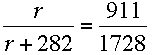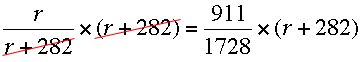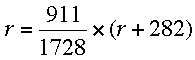SEARCH HOMEMath Central Quandaries & QueriesQuestion from Len, a parent: I am having a brain cramp or maybe I just forgot some basic math, but I am having trouble solving for "r" in your truncated cone example where r/(r+w)=r/R or r/(r+282)=911/1728. Could you refresh my memory by showing the steps to solve for "r"?Hi Len,

You have been looking at Steve's response to an art student who is constructing a truncated cone from sheet metal.

Steve gets to the equationand then says to solve for r.

I would first multiply both sides by r + 282 to eliminate the fraction on the left side. The equation then becomesSince the factor r + 282 cancels on the left you are left withAt this point you can multiply both sides by 1728 to eliminate the fraction on the right or use your calculator to find a decimal approximation of 911/1728. I'm going to multiply both sides by 1728 to obtain

1728 r = 911 × (r + 228)

and expand the right side to get

1728 r = 911 r + 911 × 228

Subtract 911 r from both sides and the equation becomes

1728 r - 911 r = 817 r = 911 × 228

and dividing both sides by 817

r = (911 × 228)/817

Now it's time for the calculator which yields

r = 314.4

I hope this helps,
HarleyMath Central is supported by the University of Regina and The Pacific Institute for the Mathematical Sciences.